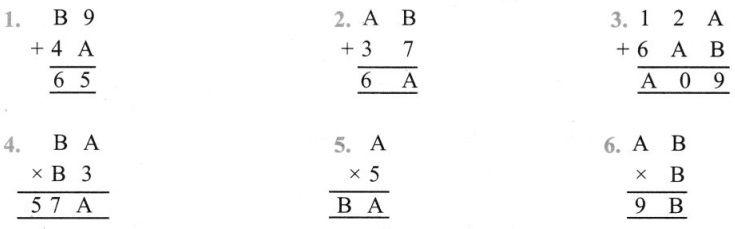# Grade 8 Playing with Numbers Worksheets

## Grade 8 Maths Playing with Numbers Multiple Choice Questions (MCQs)

1. If 31z6 is a multiple of 9 then the value of z is:
(a) 0
(b) 4
(c) 8
(d) 9

2. If 1 A × A = 9A, then what is the value of A?
(a) 3
(b) 5
(c) 6
(d) 2

3. If BA × B3 = 57A, then the value of A and B is:
(a) A = 5, B = 2
(b) A = 2, B = 5
(c) A = 5, B = 3
(d) A = 3, B = 5

4. If 2AB + AB1 = B18, then the value of A and B is:
(a) A = 5, B = 8
(b) A = 2, B = 5
(c) A = 7, B = 9
(d) A = 4, B = 7

5. If AB × 6 = BBB, then the value of A and B is:
(a) A = 7, B = 4
(b) A = 0, B=1
(c) A= 1, B = 0
(d) A = 9, B = 7

6. If 8A5 + 94A = 1A33, then what is the value of A?
(a) 0
(b) 4
(c) 8
(d) 9

7. If 2y25 is a multiple of 9, where y is a digit then the value of y is:
(a) 0
(b) 3
(c) 1
(d) 2

8. If 31y5 is a multiple of 3, where y is a digit then the value of y is:
(a) 0
(b) 1
(c) 2
(d) None of these

9. If 24x is a multiple of 11, where x is a digit then the value of x is:
(a) 0
(b) 1
(c) 2
(d) None of these

10. If 66784y is divisible by 9, where y is a digit then the value of y is:
(a) 0
(b) 3
(c) 1
(d) None of these

### Class 8 Maths Playing with Numbers Fill In The Blanks

1. A two digit numbr ab can be written in standard form as ……………… .
2. Largest 3-digit number which is divisible by 6 is ………………. .
3. If we add …………….. to 194562 then it is divisible by 5 and 10 both.
4. If we subtract ………………… from 53214 then the number so obtained is divisible by 8.
5. Smallest 4-digit number which is divisible by 7 is …………….. .

### Class 8 Maths Playing with Numbers True(T) Or False(F)

1. If a number is divisible by 3 then it is also divisible by 9.
2. If a number is divisible by 8 then it is also divisible by 4.
3. If a number is divisible by 12 then it is also divisible by both 3 and 4.
4. If sum of two consecutive odd numbers in always divisible by 4.
5. If two numbers are co-prime at least one of them must be a prime number.

### Class 8 Maths Playing with Numbers Short Answer Type Questions

1. If 35 a 6 is divisible by 3, where ‘a’ is a digit, what are the possible values of ‘a’?
2. If 67 y 19 is multiple by 9, where ‘y’ is a digit, what are the possible values of ‘y’?
3. If 15 x 2 is multiple by 4, where V is a digit, what are the possible values of ‘x’?
4. If 67 x 19 is divisible by 11, where ‘x’ is a digit, what are the possible values of ‘x’?

### Class 8 Maths Playing with Numbers Long Answer Type Questions

Find the value of letters in each of the following: